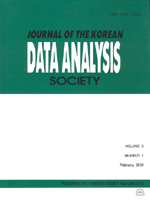상세검색
최근 검색어 전체 삭제
다국어입력
즐겨찾기0KCI등재 학술저널

# Modified Wang’s Methods Confidence Intervals for the Ratio(B) of Total Variance in Two-Factor Nested Variance Components Model

• 등재여부 : KCI등재
• 2012.12
• 2867 - 2876 (10 pages)

The confidence intervals for σ²B/(σ²A+σ²B+σ²C) are given in various forms by many authors in two-factor nested variance components model y_ijk=μ+A_i+B_ij+C_ijk. In this model, we can find out the confidence intervals for σ²A/(σ²A+σ²B+σ²C) and the confidence intervals for σ²C/(σ²A+σ²B+σ²C) by using Wang’s methods of the confidence intervals for σ²A/σ²C and the confidence intervals for σ²A/σ²B. And we can find out a confidence intervals for σ²B/(σ²A+σ²B+σ²C) by using those two confidence intervals for σ²A/(σ²A+σ²B+σ²C) and σ²C/(σ²A+σ²B+σ²C). The simulation results show that the results of the confidence intervals are not fit exactly for (1-a)% confidence intervals, and the ranges of width are wide to use for estimation and test. Thus, we gave a new modified confidence intervals for σ²B/(σ²A+σ²B+σ²C) by using those two results of confidence intervals. And by the simulation results of the modified confidence intervals for σ²B/(σ²A+σ²B+σ²C) are better than those obtained by using Wang’s methods of confidence intervals, and we can use for estimation and test.

1. Introduction

2. Two-Factor Nested Variance Components Model

3. Confidence Intervals

4. Simulation Results

5. Conclusion

References